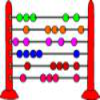#### You may also like### Which Scripts?

There are six numbers written in five different scripts. Can you sort out which is which?### A Romantic Riddle

Read this riddle and see if you can work out how the trees must be planted.### Fibonacci's Three Wishes 1

First of two articles about Fibonacci, written for students.

# Roman Numerals

##### Age 7 to 11Challenge Level
It might help if you break the numbers down into thousands, hundreds, tens and units.

What number is "XII"?

Using rule number one, and breaking the number down into tens and units:
X= ten
II= two
The two comes after the ten, and so we add two to ten, and get the number 12.